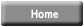Index of refraction of liquids
 Introduction: One indicator of the optical density of a material is the index of refraction value of the material. Index of refraction values (represented by the symbol n) are numerical index values which are expressed relative to the speed of light in a vacuum. The index of refraction value of a material is a number which indicates the number of times that a light wave would be in that material rather than in a vacuum. A vacuum is given an n value of 1.0000.